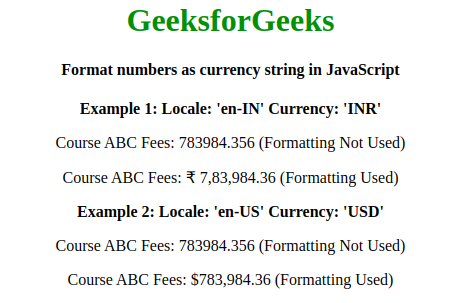Related Articles

# How to format numbers as currency string in JavaScript ?

• Difficulty Level : Medium
• Last Updated : 30 Sep, 2019

A number, represented as monetary value, creates an impact and becomes much more readable, and that’s the reason behind formatting a number as currency.
For example, a number, let’s say 100000 when represented as \$100,000.00 it becomes pretty much understand that it represents a monetary value, and the currency in which it is formatted is USD.

Different countries have different currencies, as well as different conventions to display monetary values. For example, USA follows the International Numbering System for representing USD, on the other hand, India follows Indian Numbering System for representing INR.

Syntax:

```Intl.NumberFormat('en-US', {style: 'currency', currency: 'target currency'})
.format(monetary_value);
```

Explanation:
The ‘en-INR’ and ‘en-US’ is used as the locale here, a list of all the locales can be found from here, and the currency used here is ‘INR’ and ‘USD’, but all the standard currencies are supported. Choosing a different locale and currency will format you monetary value accordingly.

Example 1:

 ```<``html``>`` ` `<``head``>``    ``<``title``>``        ``Formating number in currency string``    `````` ` `<``body``>``    ``<``center``>``        ``<``h1` `style``=``"color:green;"``>GeeksforGeeks``        ``<``h4``>``         ``Formatting 4800 as INR``        ````        ``<``script``>``            ``var format = new Intl.NumberFormat('en-INR', {``                ``style: 'currency',``                ``currency: 'INR',``                ``minimumFractionDigits: 2,``            ``});``            ``// for 4800 INR``            ``document.write(format.format(4800));``        ````    ``<``center``>```` ` ``

Output:Example 2:

 ```<``html``>`` ` `<``head``>``    ``<``meta` `charset``=``"UTF-8"``>``    ``<``meta` `name``=``"viewport"` `          ``content``=``"width=device-width, initial-scale=1"``>``    ``<``title``>Currency format``    ````    ``<``script` `src``=``"https://code.jquery.com/jquery-3.4.1.min.js"` `            ``integrity``=``"sha256-CSXorXvZcTkaix6Yvo6HppcZGetbYMGWSFlBw8HfCJo="` `            ``crossorigin``=``"anonymous"``>``    ````    `````` ` `<``body``>``    ``<``center``>``        ``<``h1` `style``=``"color:green;"``>``          ``GeeksforGeeks``        ````        ``<``h4``>``          ``Format numbers as currency string in JavaScript``        ````        ``<``b``>Example 1: Locale: 'en-IN' Currency: 'INR'``        ``<``p``>Course ABC Fees: 783984.356 (Formatting Not Used)``        ``<``p``>Course ABC Fees: ``          ``<``span` `class``=``"currency-inr"``>783984.356``          ``(Formatting Used)``        ```` ` `        ``<``b``>Example 2: Locale: 'en-US' Currency: 'USD'``        ``<``p``>Course ABC Fees: 783984.356 (Formatting Not Used)``        ``<``p``>Course ABC Fees: ``          ``<``span` `class``=``"currency-usd"``>783984.356 ``          ``(Formatting Used)``        ```` ` `        ``<``script` `type``=``"text/javascript"``>``            ``\$('.currency-inr').each(function() {``                ``var monetary_value = \$(this).text();``                ``var i = new Intl.NumberFormat('en-IN', {``                    ``style: 'currency',``                    ``currency: 'INR'``                ``}).format(monetary_value);``                ``\$(this).text(i);``            ``});``            ``\$('.currency-usd').each(function() {``                ``var monetary_value = \$(this).text();``                ``var i = new Intl.NumberFormat('en-US', {``                    ``style: 'currency',``                    ``currency: 'USD'``                ``}).format(monetary_value);``                ``\$(this).text(i);``            ``});``        ````  `````` ` `     `

Output:Note: We are using ECMAScript Internationalization API (Intl Object) for formatting purpose, that comes under the category of JavaScript Standard built-in objects and jQuery for DOM Manipulation.

My Personal Notes arrow_drop_up